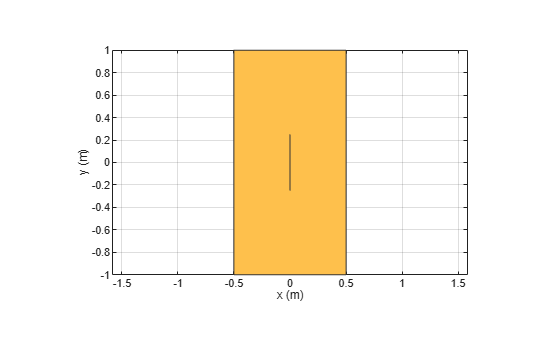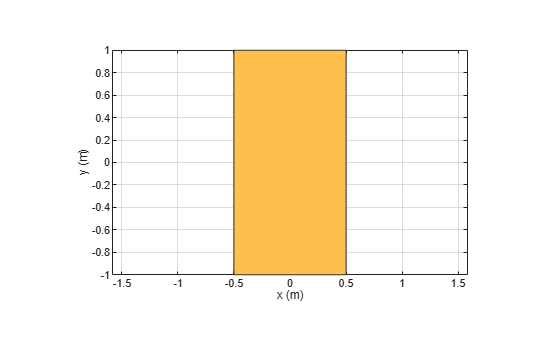removeHoles

Remove holes from shape

Description

example

s = removeHoles(shapeobject,holetol) removes holes with area less than the provided tolerance from the shape.

Examples

collapse all

Create two rectangle shapes. Change the length and the center of orientation of the second rectangle to the values shown.

rect1 = antenna.Rectangle;
rect2 = antenna.Rectangle;
rect2.Length = 1e-7;
rect2.Width = 0.5
rect2 =
Rectangle with properties:

Name: 'myrectangle'
Center: [0 0]
Length: 1.0000e-07
Width: 0.5000
NumPoints: 2

Subtract rectangle 1 from rectangle 2

rect3 = rect1-rect2
rect3 =
Polygon with properties:

Name: 'mypolygon'
Vertices: [9x3 double]

show(rect3)Remove holes from the new rectangle.

rect4 = removeHoles(rect3,1e-6)
rect4 =
Polygon with properties:

Name: 'mypolygon'
Vertices: [4x3 double]

show(rect4)Input Arguments

collapse all

Antenna shape with sliver outlier, specified as antenna.Circle, antenna.Rectangle, antenna.Polygon objects, antenna.Ellipse, or the shapes function.

Data Types: function

Hole tolerance, specified as a nonnegative scalar.

Data Types: double